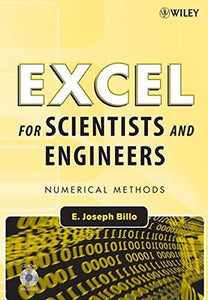Wiley-Interscience

# Excel For Scientists And Engineers: Numerical Methods

• Publish Date: 2007-04-06
• Binding: Paperback
• Author: E. Joseph Billo

Regular price \$22.65 Sale price \$141.92

Attention: For textbook, access codes and supplements are not guaranteed with used items.

Learn to fully harness the power of Microsoft Excel to perform scientific and engineering calculations

With this text as your guide, you can significantly enhance Microsoft Excel's capabilities to execute the calculations needed to solve a variety of chemical, biochemical, physical, engineering, biological, and medicinal problems. The text begins with two chapters that introduce you to Excel's Visual Basic for Applications (VBA) programming language, which allows you to expand Excel's capabilities, although you can still use the text without learning VBA. Following the author's step-by-step instructions, here are just a few of the calculations you learn to perform:

• Use worksheet functions to work with matrices
• Find roots of equations and solve systems of simultaneous equations
• Solve ordinary differential equations and partial differential equations
• Perform linear and non-linear regression
• Use random numbers and the Monte Carlo method
This text is loaded with examples ranging from very basic to highly sophisticated solutions. More than 100 end-of-chapter problems help you test and put your knowledge to practice solving real-world problems. Answers and explanatory notes for most of the problems are provided in an appendix.

The CD-ROM that accompanies this text provides several useful features:

• All the spreadsheets, charts, and VBA code needed to perform the examples from the text
• Solutions to most of the end-of-chapter problems
• An add-in workbook with more than twenty custom functions
This text does not require any background in programming, so it is suitable for both undergraduate and graduate courses. Moreover, practitioners in science and engineering will find that this guide saves hours of time by enabling them to perform most of their calculations with one familiar spreadsheet package